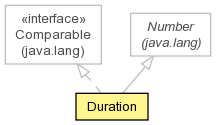## org.jboss.dna.common.math Class Duration

```java.lang.Objectjava.lang.Numberorg.jboss.dna.common.math.Duration
```
All Implemented Interfaces:
Serializable, Comparable<Duration>

`public class Durationextends Numberimplements Comparable<Duration>`A number representing an immutable duration of time. This is intended to be used in the same manner as other `Number` subclasses.

Serialized Form

Nested Class Summary
` class` `Duration.Components`
The atomic components of this duration, broken down into whole hours, minutes and (fractional) seconds.

Constructor Summary
`Duration(long nanos)`
Create a duration given the number of nanoseconds.
```Duration(long duration, TimeUnit unit)```
Create a duration and the time unit.

Method Summary
` Duration` `add(Duration duration)`
Add the supplied duration to this duration, and return the result.
` Duration` ```add(long duration, TimeUnit unit)```
Add the supplied duration to this duration, and return the result.
` int` `compareTo(Duration that)`

` double` `divide(Duration duration)`
Divide the duration by another duration to calculate the ratio.
` Duration` `divide(long denominator)`
Divide the duration by the supplied number, and return the result.
` double` `doubleValue()`

` float` `floatValue()`

` Duration.Components` `getComponents()`
Return the duration components.
` long` `getDuratinInNanoseconds()`
Return the total duration in nanoseconds.
` long` `getDuration(TimeUnit unit)`
Get the duration value in the supplied unit of time.
` BigDecimal` `getDurationInMicroseconds()`
Return the total duration in microseconds, which may contain a fraction part for the sub-microsecond component.
` BigDecimal` `getDurationInMilliseconds()`
Return the total duration in microseconds, which may contain a fraction part for the sub-microsecond component.
` BigDecimal` `getDurationInSeconds()`
Return the total duration in microseconds, which may contain a fraction part for the sub-microsecond component.
` int` `intValue()`

` long` `longValue()`

` Duration` `multiply(long scale)`
Multiply the duration by the supplied scale factor, and return the result.
` Duration` `subtract(Duration duration)`
Subtract the supplied duration from this duration, and return the result.
` Duration` ```subtract(long duration, TimeUnit unit)```
Subtract the supplied duration from this duration, and return the result.
` BigDecimal` `toBigDecimal()`

` String` `toString()`
Writes the duration in a form containing hours, minutes, and seconds, including the fractional part of the seconds.

Methods inherited from class java.lang.Number
`byteValue, shortValue`

Methods inherited from class java.lang.Object
`equals, getClass, hashCode, notify, notifyAll, wait, wait, wait`

Constructor Detail

### Duration

`public Duration(long nanos)`
Create a duration given the number of nanoseconds.

Parameters:
`nanos` - the number of nanoseconds in the duration

### Duration

```public Duration(long duration,
TimeUnit unit)```
Create a duration and the time unit.

Parameters:
`duration` - the duration in the supplied time units
`unit` - the time unit
Method Detail

### doubleValue

`public double doubleValue()`

Specified by:
`doubleValue` in class `Number`

### floatValue

`public float floatValue()`

Specified by:
`floatValue` in class `Number`

### intValue

`public int intValue()`

Specified by:
`intValue` in class `Number`

### longValue

`public long longValue()`

Specified by:
`longValue` in class `Number`

### toBigDecimal

`public BigDecimal toBigDecimal()`

```public Duration add(long duration,
TimeUnit unit)```
Add the supplied duration to this duration, and return the result.

Parameters:
`duration` - the duration to add to this object
`unit` - the unit of the duration being added; may not be null
Returns:
the total duration

### subtract

```public Duration subtract(long duration,
TimeUnit unit)```
Subtract the supplied duration from this duration, and return the result.

Parameters:
`duration` - the duration to subtract from this object
`unit` - the unit of the duration being subtracted; may not be null
Returns:
the total duration

`public Duration add(Duration duration)`
Add the supplied duration to this duration, and return the result. A null value is treated as a duration of 0 nanoseconds.

Parameters:
`duration` - the duration to add to this object
Returns:
the total duration

### subtract

`public Duration subtract(Duration duration)`
Subtract the supplied duration from this duration, and return the result. A null value is treated as a duration of 0 nanoseconds.

Parameters:
`duration` - the duration to subtract from this object
Returns:
the resulting duration

### multiply

`public Duration multiply(long scale)`
Multiply the duration by the supplied scale factor, and return the result.

Parameters:
`scale` - the factor by which the duration is to be scaled.
Returns:
the scaled duration

### divide

`public Duration divide(long denominator)`
Divide the duration by the supplied number, and return the result.

Parameters:
`denominator` - the factor by which the duration is to be divided.
Returns:
the resulting duration

### divide

`public double divide(Duration duration)`
Divide the duration by another duration to calculate the ratio.

Parameters:
`duration` - the duration that this duration is to be divided by; may not be null
Returns:
the resulting duration

### compareTo

`public int compareTo(Duration that)`

Specified by:
`compareTo` in interface `Comparable<Duration>`

### getDuratinInNanoseconds

`public long getDuratinInNanoseconds()`
Return the total duration in nanoseconds.

Returns:
the total duration in nanoseconds

### getDurationInMicroseconds

`public BigDecimal getDurationInMicroseconds()`
Return the total duration in microseconds, which may contain a fraction part for the sub-microsecond component.

Returns:
the total duration in microseconds

### getDurationInMilliseconds

`public BigDecimal getDurationInMilliseconds()`
Return the total duration in microseconds, which may contain a fraction part for the sub-microsecond component.

Returns:
the total duration in microseconds

### getDurationInSeconds

`public BigDecimal getDurationInSeconds()`
Return the total duration in microseconds, which may contain a fraction part for the sub-microsecond component.

Returns:
the total duration in microseconds

### getComponents

`public Duration.Components getComponents()`
Return the duration components.

Returns:
the individual time components of this duration

### getDuration

`public long getDuration(TimeUnit unit)`
Get the duration value in the supplied unit of time.

Parameters:
`unit` - the unit of time for the returned value; may not be null
Returns:
the value of this duration in the supplied unit of time

### toString

`public String toString()`
Writes the duration in a form containing hours, minutes, and seconds, including the fractional part of the seconds. The format is essentially `HHH:MM:SS.mmm,mmm`, where
HHH
is the number of hours written in at least 2 digits (e.g., "03")
MM
is the number of hours written in at least 2 digits (e.g., "03")
SS
is the number of hours written in at least 2 digits (e.g., "03")
mmm,mmm
is the fractional part of seconds, written in at least millisecond precision and up to microsecond precision. The comma appears if more than 3 digits are used.

Overrides:
`toString` in class `Object`
Returns:
a string representation of the duration